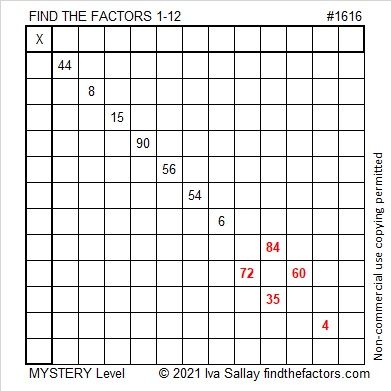# 1616 Centering the Pendulum

Contents

### Today’s Puzzle:

Centering the Pendulum is Bill Davidson’s podcast about educators and how they inspire students to learn mathematics. All of his podcasts are wonderful and more than worth the 15 minutes or so needed to listen to each one.  I am quite honored that Find the Factors is the subject of his fourth podcast, and you can listen to it here.

To mark this occasion, I’ve made a mystery level puzzle that resembles a swinging pendulum that hopefully is centered! The puzzle might or might not be a little tricky, but just use logic every step of the way, and you should be fine.Using logic, write the numbers from 1 to 12 in both the first column and the top row so that those numbers and the given clues function like a multiplication table. Have fun!

### Factors of 1616:

• 1616 is a composite number.
• Prime factorization: 1616 = 2 × 2 × 2 × 2 × 101, which can be written 1616 = 2⁴ × 101.
• 1616 has at least one exponent greater than 1 in its prime factorization so √1616 can be simplified. Taking the factor pair from the factor pair table below with the largest square number factor, we get √1616 = (√16)(√101) = 4√101.
• The exponents in the prime factorization are 4 and 1. Adding one to each exponent and multiplying we get (4 + 1)(1 + 1) = 5 × 2 = 10. Therefore 1616 has exactly 10 factors.
• The factors of 1616 are outlined with their factor pair partners in the graphic below.### More about the Number 1616:

1616 is the sum of two squares:
40² + 4² = 1616.

1616 is also the hypotenuse of a Pythagorean triple:
320-1584-1616, which is 16 times (20-99-101)
and can be calculated from 2(40)(4), 40² – 4², 40² + 4².

1616 is also the difference of two squares in three ways:
405² – 403² = 1616,
204² – 200² = 1616, and
105² – 97² = 1616.

This site uses Akismet to reduce spam. Learn how your comment data is processed.